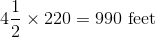## Example Questions

### Example Question #21 : Sat Mathematics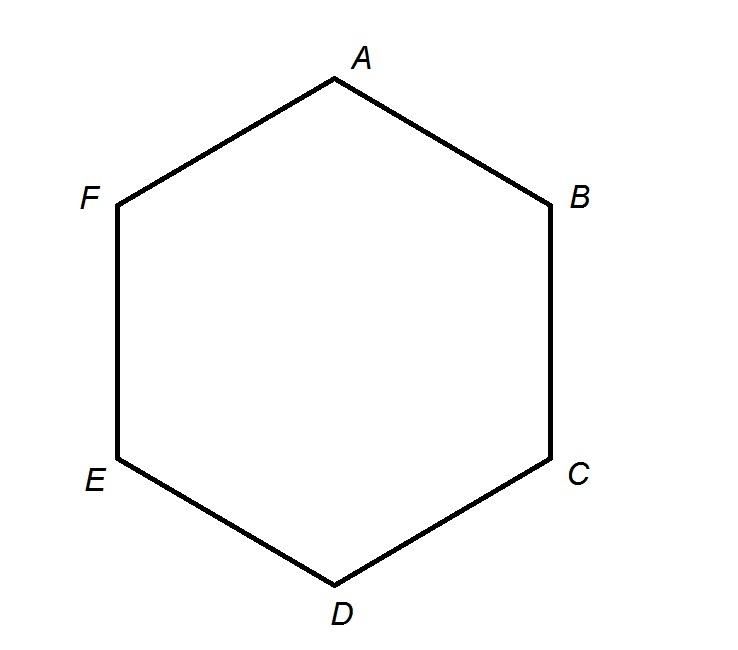The provided image represents a track in the shape of a regular hexagon with perimeter one fourth of a mile.

Teresa starts at Point A and runs clockwise until she gets halfway between Point E and Point F. How far does she run, in feet?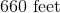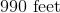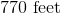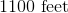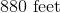Explanation:

One mile is equal to 5,280 feet; one fourth of a mile is equal to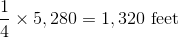Each of the six congruent sides measures one sixth of this, or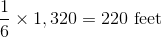Teresa runs clockwise from Point A to halfway between Point E and Point F, so she runs along four and one half sides, for a total of